# #PiDay Art from Beautiful Geometry – “Squaring the Circle”

At first glance, the circle may seem to be the simplest of all geometric shapes and the easiest to draw: take a string, hold down one end on a sheet of paper, tie a pencil to the other end, and swing it around—a simplified version of the compass. But first impressions can be misleading: the circle has proved to be one of the most intriguing shapes in all of geometry, if not the most intriguing of them all.

How do you find the area of a circle, when its radius is given? You instantly think of the formula A = πr2. But what exactly is that mysterious symbol π? We learn in school that it is approximately 3.14, but its exact value calls for an endless string of digits that never repeat in the same order. So it is impossible to find the exact area of a circle numerically. But perhaps we can do the next best thing—construct, using only straightedge and compass, a square equal in area to that of a circle?

This problem became known as squaring the circle— or simply the quadrature problem—and its solution eluded mathematicians for well over two thousand years. The ancient Egyptians came pretty close: In the Rhind Papyrus, a collection of 84 mathematical problems dating back to around 1800 BCE, there is a statement that the area of a circle is equal to the area of a square of side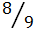of the circle’s diameter. Taking the diameter to be 1 and equating the circle’s area to that of the square, we get π(½)2 = ()2, from which we derive a value of π equal to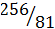≈ 3.16049—within 0.6 percent of the true value. However, as remarkable as this achievement is, it was based on “eyeballing,” not on an exact geometric construction.

Numerous attempts have been made over the centuries to solve the quadrature problem. Many careers were spent on this task—all in vain. The definitive solution—a negative one—came only in 1882, when Carl Louis Ferdinand von Lindemann (1852– 1939) proved that the task cannot be done—it is impossible to square a circle with Euclidean tools. Actually, Lindemann proved something different: that the number π, the constant at the heart of the quadrature problem, is transcendental. A transcendental number is a number that is not the solution of a polynomial equation with integer coefficients. A number that is not transcendental is called algebraic. All rational numbers are algebraic; for example,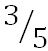is the solution of the equation 5x − 3 = 0. So are all square roots, cubic roots, and so on; for example,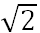is the positive solution of x2 − 2 = 0, and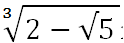is one solution of x6 − 4x3 − 1 = 0. The name transcendental has nothing mysterious about it; it simply implies that such numbers transcend the realm of algebraic (polynomial) equations.

Now it had already been known that if π turned out to be transcendental, this would at once establish that the quadrature problem cannot be solved. Lindemann’s proof of the transcendence of π therefore settled the issue once and for all. But settling the issue is not the same as putting it to rest; being the most famous of the three classical problems, we can rest assured that the “circle squarers” will pursue their pipe dream with unabated zeal, ensuring that the subject will be kept alive forever.Metamorphosis of a Circle

Metamorphosis of a Circle, shows four large panels. The panel on the upper left contains nine smaller frames, each with a square (in blue) and a circular disk (in red) centered on it. As the squares decrease in size, the circles expand, yet the sum of their areas remains constant. In the central frame, the square and circle have the same area, thus offering a computer-generated “solution” to the quadrature problem. In the panel on the lower right, the squares and circles reverse their roles, but the sum of their areas is still constant. The entire sequence is thus a metamorphosis from square to circle and back.

Of course, Euclid would not have approved of such a solution to the quadrature problem, because it does not employ the Euclidean tools—a straightedge and compass. It does, instead, employ a tool of far greater power—the computer. But this power comes at a price: the circles, being generated pixel by pixel like a pointillist painting, are in reality not true circles, only simulations of circles.1 As the old saying goes, “there’s no free lunch”—not even in geometry.

Note:

1. The very first of the 23 definitions that open Euclid’s Elements defines a point as “that which has no part.” And since all objects of classical geometry—lines, circles, and so on—are made of points, they rest on the subtle assumption that Euclidean space is continuous. This, of course, is not the case with computer space, where Euclid’s dimensionless point is replaced by a pixel—small, yet of finite size—and space between adjacent pixels is empty, containing no points.